Name:    Electrons in Atoms Practice Test

True/False
Indicate whether the statement is true or false.

1.

When an atom loses electrons, its radius gets smaller.

2.

Although the precise location of an electron cannot be determined, it is possible to determine the probability that an electron will occupy a certain region around the nucleus.

3.

An atom that gains an electron will form a positive ion.

4.

As the amount of energy carried by a wave decreases, its wavelength increases.

5.

The electronegativity of elements increases from left to right and from top to bottom on the periodic table.

6.

Elements in the same family tend to have the same number of valence electrons.

7.

Valence electrons refers to the total number of electrons that exist in the orbitals that occupy the outermost energy level of an atom.

8.

The unit for measuring the energy of a wave is Hertz (1/sec).

9.

The maximum number of allowed valence electrons in any atom is 8.

10.

Six electrons can exist in a p-orbital.

11.

The radius of an atom is defined as half the distance between the nuclei of two adjacent or bonded atoms of that element.

12.

The number of electrons in an orbital depends on the orbital’s shape.

13.

[Kr]5s24d105p5 is the correct electron configuration for the element Bromine, Br.

14.

An element can be identified by the characteristic bright-line atomic emission spectrum it can produce.

15.

An p orbital is in the shape of a dumbbell.

16.

The photoelectric effect describes the emission of electrons from a metal’s surface when light at a specific frequency shines on it.

Multiple Choice
Identify the choice that best completes the statement or answers the question.

17.

A photon is emitted from an atom with an energy of 4.25 x 10-19 J. What is the wavelength of the photon?
 a. 273 nm b. 425 nm c. 642 nm d. 467 nm

18.

What is the frequency of a light wave with a wavelength of 680 nm? (convert to meters first!)
 a. 2.04 s c. 4.41 x 1014 Hz b. 2.04 x 109 Hz d. 2.27 x 10-15 Hz

19.

Which type of orbital is shown?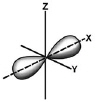a. p b. d c. f d. s

20.

Emission of light from an atom occurs when an electron
 a. jumps from a lower to a higher energy level. b. moves within its atomic orbital. c. drops from a higher to a lower energy level. d. falls into the nucleus.

21.

How does the energy of an electron change when the electron moves closer to the nucleus?
 a. It decreases. b. It doubles. c. It stays the same. d. It increases.

22.

Which is the correct number of valence electrons in the element Gallium (Ga)?
 a. 31 b. 3 c. 1 d. 13

23.

Which is defined as the energy required to remove an electron from an atom of an element in the gaseous state? (doesn’t want to give up any!)
 a. ionization energy c. electronegativity b. law of octets d. ionic radius

24.

What is the maximum number of f orbitals in any single energy level in an atom?
 a. 7 b. 1 c. 5 d. 325.

Which region contains elements with two valence electrons?
 a. A b. B c. C d. D

26.

Which region contains elements with an electron configuration that ends with p1?
 a. A b. B c. C d. D

27.

Which of the following factors contributes to the increase in ionization energy from left to right across a period?
 a. an increase in the shielding effect b. fewer electrons in the highest occupied energy level c. an increase in the number of protons, the effective nuclear charge d. an increase in the size of the nucleus

28.

What element in the second period has the largest atomic radius?
 a. potassium b. neon c. lithium d. carbon

29.

When an electron moves from a lower to a higher energy level, the electron
 a. moves closer to the nucleus. b. always doubles its energy. c. absorbs a quantum of energy. d. absorbs a continuously variable amount of energy.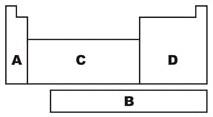30.

Which region is referred to as the f-block on the diagram?
 a. A b. B c. C d. D

31.

Which region is referred to as the s-block on the diagram?
 a. A b. B c. C d. D

32.

What is the maximum number of d orbitals in a principal energy level?
 a. 2 b. 5 c. 3 d. 1

33.

Which is the correct number of valence electrons in the element Carbon?
 a. 6 b. 2 c. 16 d. 4

34.

Which element has the electron configuration 1s22s22p63s23p64s23d4?
 a. Cr b. Ti c. Se d. S

35.

What types of atomic orbitals are in the third principal energy level?
 a. s and p only b. p and d only c. s, p, d, and f d. s, p, and d only36.

Which element has an electron configuration that ends in the fourth energy level?
 a. A b. B c. C d. D

37.

Which element has full valence shell?
 a. A b. B c. C d. D

38.

The atomic number of an element is the total number of which particles in the nucleus?
 a. electrons c. protons and electrons b. protons d. neutrons

39.

Which correctly describes elements in the same group?
 a. They must be in the same state of matter. b. They have the same number of valence electrons. c. They have the same atomic radius. d. They have electrons in the same outermost energy level.

40.

The atomic emission spectra of a sodium atom on Earth and of a sodium atom in the sun would be
 a. the same. b. different from each other. c. the same as those of several other elements. d. the same as each other only in the ultraviolet range.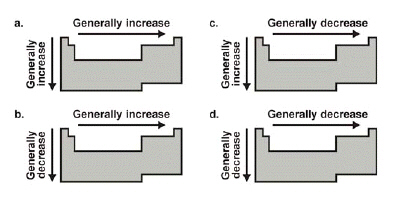41.

Which diagram correctly depicts the general trend in first ionization energy?
 a. a b. b c. c d. d

42.

Which diagram correctly depicts the trend in atomic radius?
 a. a b. b c. c d. d

43.

What is the shape of the 3p atomic orbital?
 a. sphere b. donut c. bar d. dumbbell

44.

Each period in the periodic table corresponds to a(n) ____.
 a. orbital c. suborbital b. principal energy level d. sublevel

45.

Identify the period and group numbers of the element with the electron configuration. [Ne]3s23p4
 a. Period 2, Group 16 c. Period 3, Group 4 b. Period 3, Group 16 d. Period 2, Group 4

46.

What is the charge of a cation?
 a. a negative charge b. a positive charge c. no charge

47.

Which is the most important characteristic in detemining an element’s chemical properties?
 a. the number of valence electrons it contains b. which period it is found in c. its outermost energy level d. the number of protons and neutrons in its nucleus

48.

How does atomic radius change from left to right across a period in the periodic table?
 a. It tends to decrease. b. It tends to increase. c. It first decreases, then increases. d. It first increases, then decreases.

49.

Which of the following elements has the largest atomic radius?
 a. F b. Li c. C d. Br

50.

Which is a transition element with five d-block electrons in energy level 4?
 a. Manganese (Mn) c. Technicium (Tc) b. Niobium (Nb) d. Renium (Re)

51.

What is the electron configuration of potassium, K?
 a. 1s2s2p3s3pc. 1s2s3s3p3db. 1s2s2p3s3p4sd. 1s2s2p3s3p4s52.

What is the maximum number of electrons in the second principal energy level?
 a. 32 b. 8 c. 2 d. 18

53.

What element has the electron configuration 1s2s2p3s3p?
 a. Si b. Se c. Ag d. N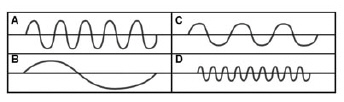54.

Which diagram shows a wave with the highest frequency?
 a. A b. B c. C d. D

55.

How many energy sublevels are in the second principal energy level?
 a. 1 b. 4 c. 3 d. 2

56.

Which of the following elements has the smallest first ionization energy?
 a. Ca b. Na c. Mg d. K

57.

How many half-filled orbitals are in a bromine atom?
 a. 3 b. 1 c. 4 d. 2

58.

Which rule for the filling of orbitals in the element Phosphorus is being violated?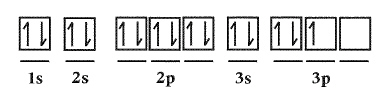a. Aufbau Principle c. No rules are violated b. Hund’s Rule d. Pauli Exclusion Principle

59.

How does atomic radius change from top to bottom in a group in the periodic table?
 a. It tends to increase. b. It first decreases, then increases. c. It tends to decrease. d. It first increases, then decreases.

60.

What is the maximum number of orbitals in the p sublevel?
 a. 2 b. 4 c. 3 d. 5

61.

Which rule for the filling of orbitals by electrons in the element Magnesium is being violated? Justify your answer.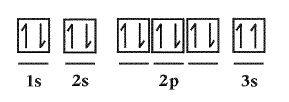a. No rules are violated c. Hund’s Rule b. Aufbau Principle d. Pauli Exclusion Principle

62.

Which of the following statements correctly compares the relative size of an ion to its neutral atom?
 a. The radius of a cation is identical to the radius of its neutral atom. b. The radius of an anion is identical to the radius of its neutral atom. c. The radius of an anion is greater than the radius of its neutral atom. d. The radius of a cation is greater than the radius of its neutral atom.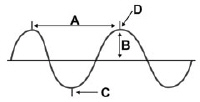63.

Which label identifies the wavelength of the wave shown?
 a. A b. B c. C d. D

64.

What is the element with the lowest electronegativity value?
 a. Cs b. F c. Ca d. He

65.

Which rule is being violated?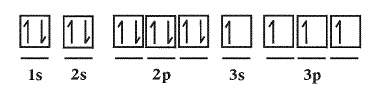a. Hund’s Rule c. Aufbau Principle b. Pauli Exclusion Principle d. No rules are violated

66.

What are quanta of light called?
 a. muons b. excitons c. photons d. charms

67.

What is the element with the highest electronegativity value?
 a. Ca b. Cl c. Cs d. Br

68.

How are the frequency and wavelength of light related?
 a. Frequency equals wavelength divided by the speed of light. b. Wavelength is determined by dividing frequency by the speed of light. c. They are directly proportional to each other. d. They are inversely proportional to each other.

69.

Use Plank’s constant, h= 6.626 ´ 10-34, to solve the following:
What is the amount of energy carried by a photon that has a frequency(v) of 5.71 x 1014 Hz?
E=hv
 a. 525 nm c. 1.14 x 10-8 J b. 3.78 x 10-19 J d. 8.62 x 1047 J/s

70.

What is the next atomic orbital in the series 1s, 2s, 2p, 3s, 3p, 4s?
 a. 2d b. 4s c. 3f d. 3d